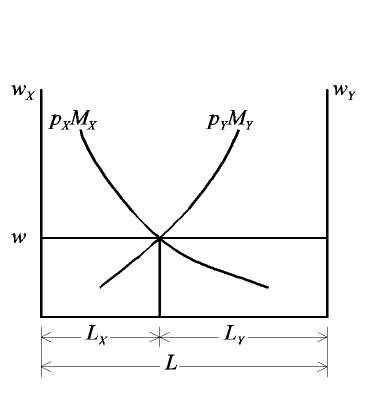# Specific Factors Model

 Pass mouse over underlined tags to change figure:

Increase in Price
of X    of Y

Increase in Endowment of
Specific Factor
in X    in Y

Increase in Endowment of
Labor## Key:

 wX, wY Wages of labor in industries, X, Y  (both equal to w in equilibrium) pX, pY Prices of X, Y MX, MY Marginal product of labor in industries X, Y LX, LY Labor employed in industries X, Y   (sum to labor endowment L in equilibrium)

## Explanation:

The economy has two sectors and three factors. Each sector has a specific factor that is confined to it, and each also employs labor, which is perfectly mobile between them. The diagram depicts the labor market, the fixed supply of labor (the labor endowment) being its width, and the two sectors' demands for labor drawn as functions of the wage from opposite corners. Demand for labor in each sector is given by a curve the height of which is the value of labor's marginal product in the sector: pM. Equilibrium is at the intersection of these two curves, determining both the common wage and the allocation of labor between the sectors.
 Effects of Changing Prices A rise in the price of either good shifts its sector's labor demand curve proportionally upward. This causes its intersection with the unchanged labor demand curve of the other sector to move up along that curve, increasing both the equilibrium common wage and employment in the sector where the price has risen. Implicit in this is that output rises in the sector with the higher price and falls in the other sector. Also, since the wage rises by less than the upward shift of the curve (the heavy arrow in the figure, showing the size of the price increase), it follows that the nominal wage of labor rises relative to the unchanged price but falls relative to the increased price, implying that the effect on the real wage is, from this information, ambiguous. Also implicit in the figure are the effects on real returns to specific factors, which respond to changes in labor employment by rising in the expanding sector and falling in the contracting sector. Effects of Changing Endowments of Specific Factors An increase in the endowment of a specific factor in a sector makes labor in that sector more productive. Assuming constant returns to scale, the marginal product of labor depends only on the ratio of factors, and therefore the marginal product curve shifts horizontally by the percentage change in the specific factor, as shown. Like the price increases above, this raises the equilibrium employment in the sector with more of the specific factor, and raises the equilibrium nominal wage in both. Output rises in the expanding sector, due now to the increases in both factors, while output in the other sector contracts. Since prices in this case are fixed, the rise in nominal wage is also an increase in real terms. The real return to the non-expanded specific factor goes up, since it depends on its ratio to employed labor. The real return to the expanded specific factor goes down, analogously, since employment of labor in that sector expands by less than the increase in the specific factor (shown again by the heavy arrow in the figure). Effects of Changing Endowment of Labor An increase in endowment of labor expands the horizontal dimension of the figure. Holding its left side fixed, the right side moves to the right by the amount of the increased endowment, and since the Y-sector labor demand is drawn relative to the vertical axis on the right, it shifts horizontally by the same absolute amount. The result is a fall in the equilibrium wage and a rise in employment of labor (and thus both output and returns to specific factors) in both sectors.